## Chapter 8 Quadratic Equations R.D. Sharma Solutions for Class 10th Math Exercise 8.7

1. Find the consecutive numbers whose squares have the sum 85.

Solution2. Divide 29 into two parts so that the sum of the squares of the parts is 425.

Solution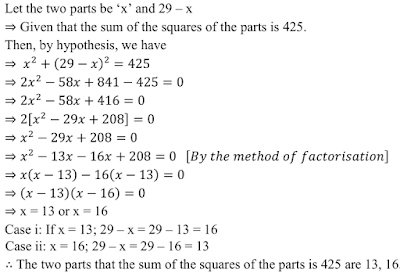3. Two squares have sides x cm and (x + 4)cm. The sum of this areas is 656 cm 2 . Find the sides of the squares.

Solution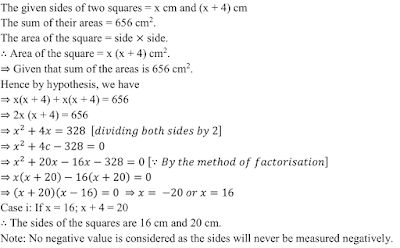4. The sum of two numbers is 48 and their product is 432. Find the numbers .

Solution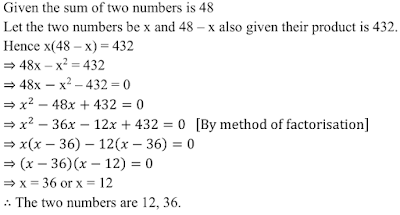5. If an integer is added to its square, the sum is 90. Find the integer with the help of quadratic equation.

Solution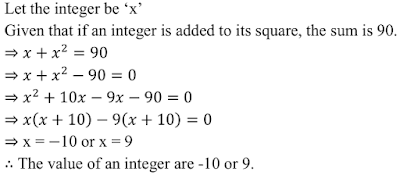6. Find the whole numbers which when decreased by 20 is equal to 69 times the reciprocal of the members.

Solution7. Find the two consecutive natural numbers whose product is 20.

Solution8. The sum of the squares of the two consecutive odd positive integers as 394. Find them.

Solution9. The sum of two numbers is 8 and 15 times the sum of their reciprocals is also 8. Find the numbers.

Solution10. The sum of a numbers and its positive square root is 6/25 . Find the numbers.

Solution11. The sum of a number and its square is 63/4. Find the numbers.

Solution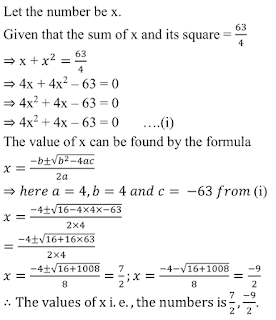12. There are three consecutive integers such that the square of the first increased by the product of the first increased by the product of the others the two gives 154. What are the integers ?

Solution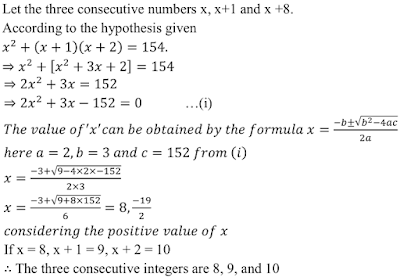13. The product of two successive integral multiples of 5 is 300. Determine the multiples.

Solution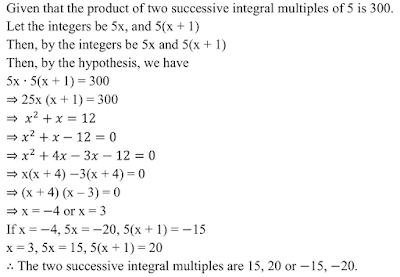14. The sum of the squares of two numbers as 233 and one of the numbers as 3 less than twice the other number find the numbers.

Solution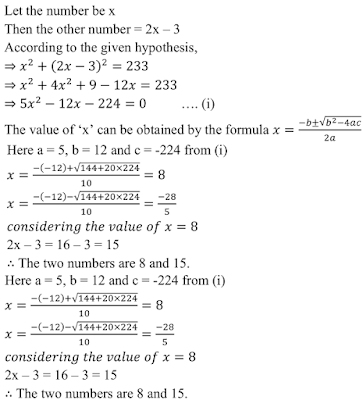15. Find the consecutive even integers whose squares have the sum 340.

Solution16. The difference of two numbers is 4. If the difference of their reciprocals is 4/21. Find the numbers.

Solution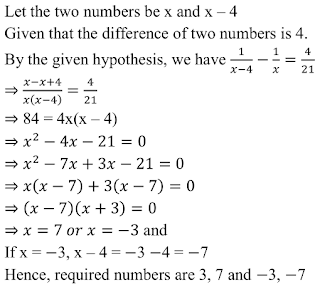17. Let us find two natural numbers which differ by 3 and whose squares have the sum 117.

Solution18. The sum of the squares of three consecutive natural numbers as 149. Find the numbers.

Solution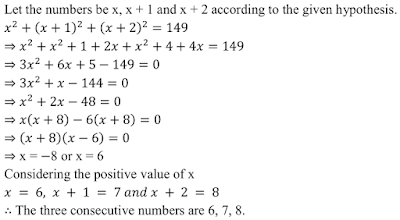19. The sum of two numbers is 16. The sum of their reciprocals is 1/3 . Find the numbers.

Solution20. Determine two consecutive multiples of 3, whose product is 270.

Solution21. The sum of a number and its reciprocal is 17/4. Find the number.

Solution22. A two-digit number is such that the products of its digits is 8. When 18 is subtracted from the number, the digits interchange their places. Find the number ?

Solution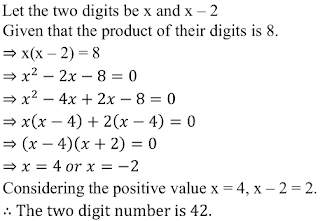23. A two digits number is such that the product of the digits is 12. When 36 is added to the number, the digits inter change their places determine the number

Solution24. A two digit number is such that the product of the digits is 16. When 54 is subtracted from the number the digits are interchanged. Find the number .

Solution25. Two numbers differ by 3 and their product is 504. Find the number .

Solution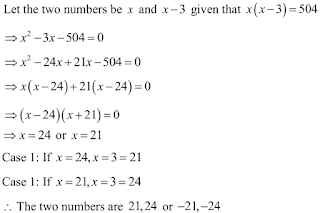26. Two number differ by 4 and their product is 192. Find the numbers ?

Solution27. A two-digit number is 4 times the sum of its digits and twice the product of its digits. Find the numbers .

Solution28. The differences of the square of two positive integers is 180 . The square of the smaller number is 8 times the larger , find the numbers .

Solution29. The sum of two numbers is 18. The sum of their reciprocals is 1/4. Find the numbers .

Solution30. The sum of two numbers and . a and b is 15. and the sum of their reciprocals 1/a and 1/b is 3/10. Find the numbers a and b.

Solution31. The sum of two numbers is 9. The sum of their reciprocals is 1/2 . Find the numbers.

Solution32. Three consecutive positive integers are such that the sum of the square of the first and the product of other two is 46. Find the integers

Solution33. The difference of squares of two numbers is 88. If the larger number is 5 less than twice the smaller number, then find the two numbers .

Solution34. The difference of square of two numbers is 180 . the square of the smaller number is 8 times the large numbers find two numbers

Solution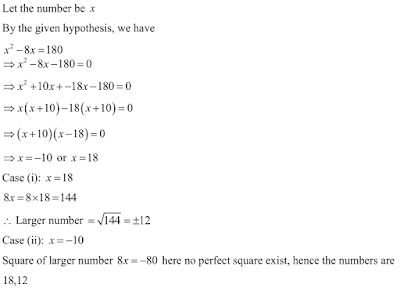35. Find two consecutive odd positive integers, sum of whose squares is 970 .

Solution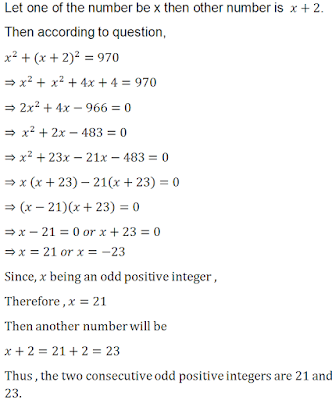36. The differences of two natural numbers is 3 and the difference of their reciprocals is 3/28 .

Solution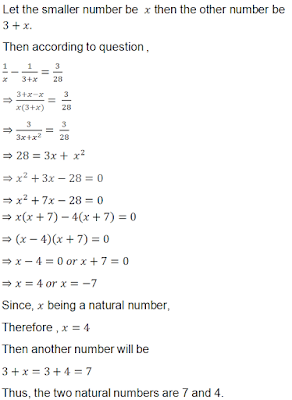37. The sum of the squares of two consecutive odd numbers is 394. Find the numbers .

Solution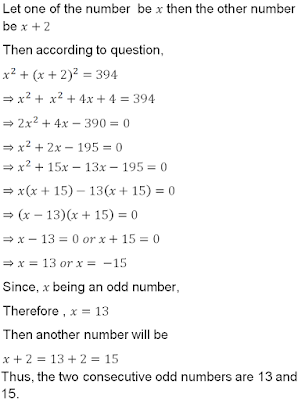38. The sum of the square of two consecutive multiples of 7 is 637. Find the multiples Sol.

Solution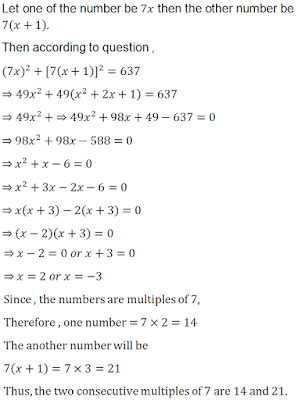39. The sum of the squares of two consecutive even numbers is 340. Find the numbers .

Solution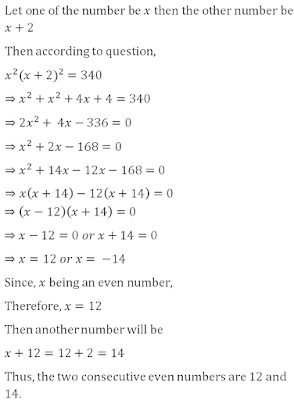40. The numerator of a fraction is 3 less than the denominator. If 2 is added to both the numerator and the denominator, then the sum of the new fraction and the original fraction is 29/20. Find the original fraction.

Solution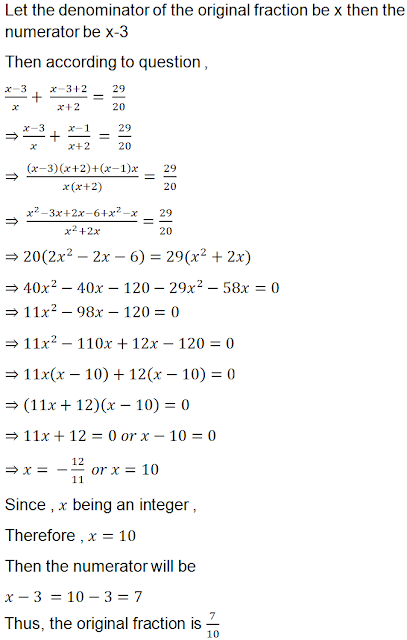41. Find a natural number whose square diminished by 84 is equal to thrice of 8 more than the given number .

Solution42. A natural number when increased by 84 equals to 160 times its reciprocal . Find the number.

Solution